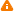Numerical Analysis and Computer Graphics - AP445001
Title: Numerical Analysis and Computer Graphics Department of Mathematics, Informatics and Cybernetics (446) Faculty of Chemical Engineering from 2022 both 0 0 3/0, other [HT] winter:unknown / unknown (unknown)summer:unknown / unknown (unknown)unlimited English full-time full-time doctoral http://uprt.vscht.cz/prochazka/pedag/lectures/SP0_MATLAB_2006EN.pdf course is intended for doctoral students onlycan be fulfilled in the futureyou can enroll for the course in winter and in summer semester
Guarantor: Švihlík Jan doc. Ing. Ph.D. D445008, P445001
 Annotation - ---CzechEnglish
Last update: Pátková Vlasta (08.06.2018)
The subject presents computational and visualization tools of the MATLAB / SIMULINK environment and its use for numeric and symbolic solution of selected computational problems. A special attention is paid to problems of data analysis, solution of linear algebraic equations, linear and nonlinear approximation, solution of nonlinear equations, to methods of numeric interpolation, derivation and integration and to the solution of difference equations. Further topis will include fundamentals of modelling and data visualization. Selected case studies will be devoted to applications of computational methods for processing of engineering and biomedical signals and images.
 Aim of the course - ---CzechEnglish
Last update: Pátková Vlasta (08.06.2018)

Students will obtain knowledge in

(i) algorithmic approach to engineering problems, programming and visualization in the MATLAB computational environment,

(ii) the use of symbolic mathematics,

(iii) block oriented data processing in the SIMULINK environment,

(iv) computational processing of selected numerical methods with applications

(v) application of numerical methods for real data processing

 Descriptors - ---CzechEnglish
Last update: Pátková Vlasta (08.06.2018)

Numerical and statistical methods, data processing, symbolic mathematics, algorithms, MATLAB programming, visualization

 Literature - ---CzechEnglish
Last update: Jahoda Milan doc. Dr. Ing. (29.11.2018)

R.L. Burden: Numerical Analysis, Brooks / Cole, 2001

S.C. Chapra: Applied Numerical Metods with Matlab for Engineers and Scientists, McGraw Hill, 2008

WIKIBOOK: MATLAB Programming, https://en.wikibooks.org/wiki/MATLAB_Programming, 2018

 Learning resources - ---CzechEnglish
Last update: Jahoda Milan doc. Dr. Ing. (29.11.2018)

http://uprt.vscht.cz/prochazka/pedag/lectures/SP0_MATLAB_2006EN.pdf

 Teaching methods - ---CzechEnglish
Last update: Pátková Vlasta (08.06.2018)

Lectures and practical verification of proposed algorithms in the computer laboratory.

 Requirements to the exam - ---CzechEnglish
Last update: Pátková Vlasta (08.06.2018)

Participation on the final colloquium with the presentation and discussion of a selected research topic.

 Syllabus - ---CzechEnglish
Last update: Pátková Vlasta (08.06.2018)

1. Computational and visualization system MATLAB, algorithmic and programming tools

2. Functions, object graphics, data structures, symbolic mathematics in MATLAB

3. Block oriented computational tools, modelling in the SIMULINK environment

4. Numeric a symbolic methods of linear algebra

5. Linear and nonlinear approximation of functions, the least square method, gradient methods

6. Solution of nonlinear equations and their systems

7. Numeric and symbolic methods of interpolation, derivation and integration

8. Solution of differencial equations

9. The boundary method and the shooting method for solution of differential equations

10. Computational intelligence in data analysis

11. The use of computational methods for processing of engineering and biomedical signals

12: CASE STUDY 1: Aproximation and statistical analysis in data processing

13: CASE STUDY 2: Solution of nonlinear equations

14: CASE STUDY 3: Modeling in the block oriented SIMULINK environment

 Entry requirements - ---CzechEnglish
Last update: Pátková Vlasta (08.06.2018)

Fundamentals of computer intelligence

 Registration requirements - ---CzechEnglish
Last update: Pátková Vlasta (08.06.2018)

Mathematics 1, 2

 Course completion requirements - ---CzechEnglish
Last update: Pátková Vlasta (08.06.2018)

Úspěšné absolvování závěrečného kolokvia s presentací a diskusí vybraného odborného tématu.